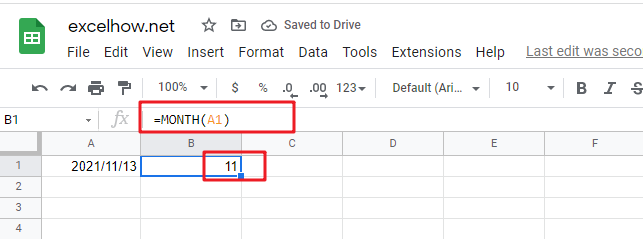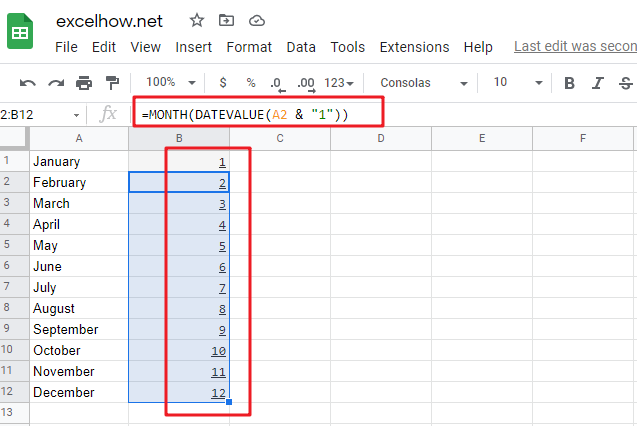This post will guide you how to use Google Sheets MONTH function with syntax and examples.

Description

The Google Sheets MONTH function returns the month of a date represented by a serial number. And the month is an integer number from 1 to 12. And you can use the MONTH function to get a month component from a given date value. And you can feed the month number to another formula.

The MONTH function can be used to extract the month from a given date as a number between 1 to 12 in google sheets. The purpose of this function is to get MONTH as a number from a date and its returned values is a number between 1 and 12.

The MONTH function is a build-in function in Google Sheets and it is categorized as a Date function.

Syntax

The syntax of the MONTH function is as below:

=MONTH (date)

Where the MONTH function argument is:

• Date -This is a required argument. A valid date value that contains the month component you want to extract. Dates should be entered by using the DATE function, or as results of other formulas or functions. For example, use DATE (2021,3,15) for the 15th day of March, 2021.

Note:

• You need to make sure that the Date argument is a cell reference that containing a date, or a function which returns a date value or a date serial number.
• If Date argument is not recognized, MONTH function will return #VALUE! Error message.
• If Date argument is supplied as a date number that is out of range, MONTH function will return #NUM! error message.
• You can use the MONTH function to extract a month component from a given date, or using this function to feed a month number into another function to create a more complex formula like the DATE function.
• Date argument can be supplied to the MONTH function as a date text string (e.g. “2021/11/13” or “Nov-11-2021”).  Or creating a date value with separate year, month, and day by using the DATE function.

The below examples will show you how to use google sheets MONTH Function to return an integer number that representing the month component of a given date.

1# get month of the date in Cell A1, enter the following formula in Cell B1.

=MONTH(A1)2# convert Month name to number, type the following formula in Cell B1.

You should use the DATEVALUE function to convert a date stored as text to a serial number. Then feed the result into the MONTH function to extract a month number from date.

=MONTH(DATEVALUE(A1 & "1"))Related Posts

This post will guide you how to use Google Sheets FLOOR function with syntax and examples. Description The Google Sheets FLOOR function returns a number rounded down to the nearest multiple of significance. So it will return a rounded number. ...

This post will guide you how to use Google Sheets EXP function with syntax and examples. Description The Google Sheets EXP function returns e raised to the power of a number.  The constant e is equal to 2.71828182845904, and it ...

This post will guide you how to use Google Sheets FACT function with syntax and examples. Description The Google Sheets FACT function returns the factorial of a given number.  The factorial of a given number is equal to 1*2*3…* number. ...

This post will guide you how to use Google Sheets EVEN function with syntax and examples. Description The Google Sheets EVEN function rounds a given number up to the nearest even integer. You can use the EVEN function to return ...

This post will guide you how to use Google Sheets COUNTBLANK function with syntax and examples. Description The Google Sheets COUNTBLANK function use to count the number of empty cells in a range of cells. You can use the COUNTBLANK ...

This post will guide you how to use Google Sheets DEGREES function with syntax and examples. Description The Google Sheets DEGREES function converts radians into degrees. And it will return a numeric value. For examples, if you pass the PI() function into ...

This post will guide you how to use Google Sheets COSH function with syntax and examples. Description The Google Sheets COSH function returns the hyperbolic cosine of a given angle. The purpose of this function is used to get hyperbolic ...